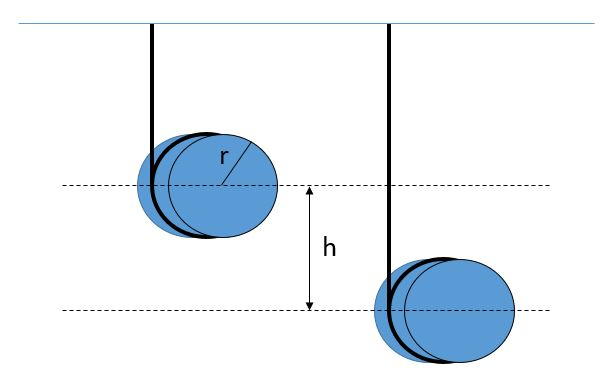PH 212

Rolling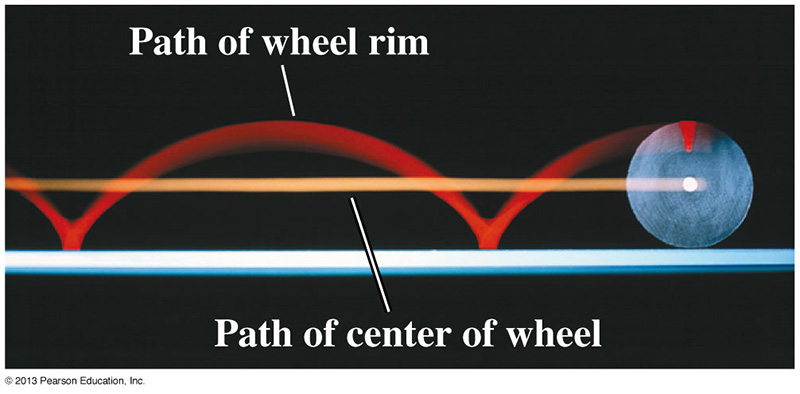Rolling is a combination of rotational motion about an axis and translational motion of the object. In the image above, a point on the outer rim of the wheel traces out a cycloid shape. The distance between the points where the cycloid touches the ground equals the circumference of the wheel. This distance also equals the distance that the center of mass of the wheel traveled in one rotational period.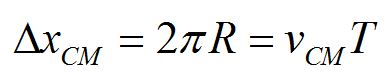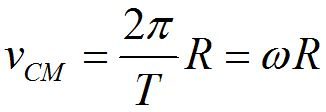The kinetic energy of an object that rolls without slipping is the sum of the translational and rotational kinetic energies of the object.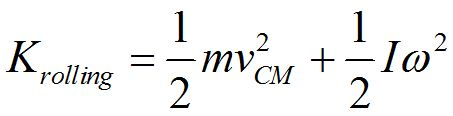A hoop, cylinder and sphere, all with the same mass, roll down a slope. Which object reaches the bottom first?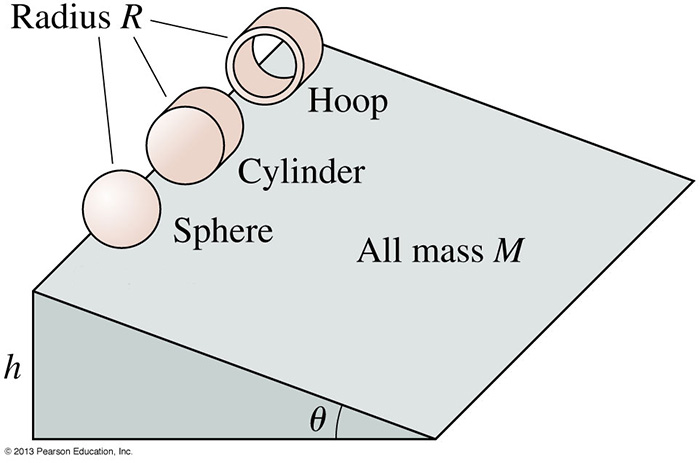Consider a cylinder of radius r and mass m, with a string wound around it, starting from rest.

How what is the velocity of the center of mass of the cylinder when it has fallen a distance h?